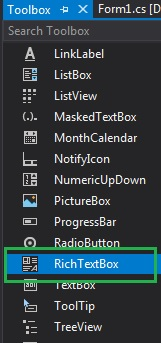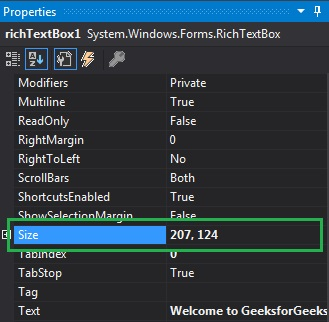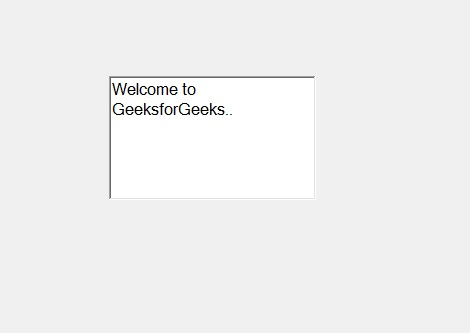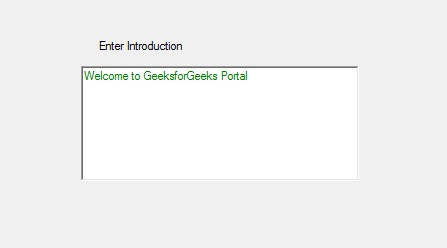# How to set the Size of the RichTextBox in C#?

• Last Updated : 02 Aug, 2019

In C#, RichTextBox control is a textbox which gives you rich text editing controls and advanced formatting features also includes a loading rich text format (RTF) files. Or in other words, RichTextBox controls allows you to display or edit flow content, including paragraphs, images, tables, etc. In RichTextBox, you set the size of the RichTextBox control using Size Property. With the help of this property, you can set both the height and width of the RichTextBox in pixels. You can set this property in two different ways:

1. Design-Time: It is the easiest way to set the size of the RichTextBox as shown in the following steps:

• Step 1: Create a windows form as shown in the below image:
Visual Studio -> File -> New -> Project -> WindowsFormApp• Step 2: Next, drag and drop the RichTextBox control from the toolbox to the form as shown in the below image:• Step 3: After drag and drop you will go to the properties of the RichTextBox and set the size of the RichTextBox as shown in the below image:Output:2. Run-Time: It is a little bit trickier than the above method. In this method, you can set the size of the RichTextBox control programmatically with the help of given syntax:

`public System.Drawing.Size Size { get; set; }`

Here, the Size indicates both height and width in pixels. The following steps show how to set the size of the RichTextBox dynamically:

• Step 1: Create a RichTextBox using the RichTextBox() constructor is provided by the RichTextBox class.
```// Creating a RichTextBox
RichTextBox rbox = new RichTextBox();
```
• Step 2: After creating RichTextBox, set the Size property of the RichTextBox provided by theRichTextBox class.
```// Setting the size
rbox.Size = new Size(278, 115);
```
• Step 3: And last add this RichTextBox control to the form using the following statement:
```// Adding this control to the form
```

Example:

 `using` `System;``using` `System.Collections.Generic;``using` `System.ComponentModel;``using` `System.Data;``using` `System.Drawing;``using` `System.Linq;``using` `System.Text;``using` `System.Threading.Tasks;``using` `System.Windows.Forms;`` ` `namespace` `WindowsFormsApp51 {`` ` `public` `partial` `class` `Form1 : Form {`` ` `    ``public` `Form1()``    ``{``        ``InitializeComponent();``    ``}`` ` `    ``private` `void` `Form1_Load(``object` `sender, EventArgs e)``    ``{``        ``// Creating and setting the``        ``// properties of the label``        ``Label lb = ``new` `Label();``        ``lb.Location = ``new` `Point(251, 70);``        ``lb.Text = ``"Enter Introduction"``;`` ` `        ``// Adding this label in the form``        ``this``.Controls.Add(lb);`` ` `        ``// Creating and setting the``        ``// properties of RichTextBox``        ``RichTextBox rbox = ``new` `RichTextBox();``        ``rbox.Location = ``new` `Point(236, 97);``        ``rbox.Size = ``new` `Size(278, 115);``        ``rbox.BorderStyle = BorderStyle.FixedSingle;``        ``rbox.ForeColor = Color.Green;``        ``rbox.Text = ``"Welcome to GeeksforGeeks Portal"``;`` ` `        ``// Adding this RichTextBox``        ``// in the form``        ``this``.Controls.Add(rbox);``    ``}``}``}`

Output:My Personal Notes arrow_drop_up